# Two Level Implementation of Logic Gates

• Last Updated : 22 Feb, 2022

The term “two-level logic” refers to a logic design that uses no more than two logic gates between input and output. This does not mean that the entire design will only have two logic gates, but it does mean that the single path from input to output will only have two logic gates.

In two-level logic, irrespective of the total number of logic gates, the maximum number of logic gates that can be cascaded between any input and output is two. The outputs of first-level logic gates are connected to the inputs of second-level logic gates in this configuration.

Example of two-level logic implementation: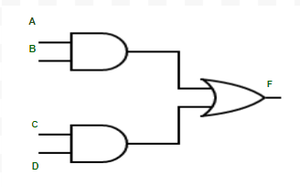An Example of Two-level implementation

We explore four logic gates in two-level logic implementation: AND Gate, OR Gate, NAND Gate, and NOR Gate. There are a total of 16 two-level logic combinations if we choose one of these four gates at the first level and one at the second level. These are

```AND-AND,  AND-OR,  AND-NAND,  AND-NOR,
OR-AND,   OR-OR,   OR-NAND,   OR-NOR,
NAND-AND, NAND-OR, NAND-NAND, NAND-NOR,
NOR-AND,  NOR-OR,  NOR-NAND,  NOR-NOR.```

Each two-level combination implements a separate logic function. These 16 combinations are divided into two categories.

1. Degenerative form  of logic gate Combination
2. Non-Degenerative form of logic gate Combination

### Degenerative form:

Degenerative form occurs when the output of a two-level logic realization can be achieved with only one logic gate. The advantage of degenerative form is that the number of inputs of single Logic gate increases which results in the increment of fan-in of logic gates.

In those 16 combinations, there are 8 degenerate forms. Below are instances of each of these degenerate types.

### AND-AND implementation:

Because the entire function results in an AND function of all the inputs, this AND-AND gate combination is a degenerate form.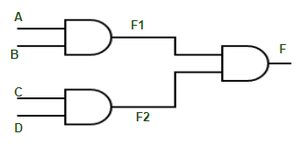AND-AND Implementation

The outputs of first-level logic gates: F1=AB and F2=CD. These outputs are applied as inputs of the second level, so the output of the second level is F=F1F2, which means F=ABCD.

### OR-OR implementation:

The output of an OR-OR gate combination is the Logic Function OR. With this combination, the OR function can be implemented with several inputs.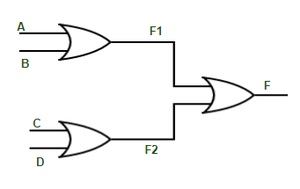OR-OR implementation

The outputs of first-level logic gates: F1=A+B and F2=C+D. These outputs are applied as inputs of the second level, so the output of the second level is F=F1+F2  which means F=A+B+C+D.

### AND-NAND implementation:

AND gates are present in the first level of this logic implementation, while NAND gates are present in the second level. An example of AND-NAND logic realization is shown in the diagram below.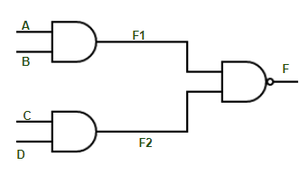AND-NAND Implementation

The outputs of first-level logic gates: F1=AB and F2=CD. These outputs are applied as inputs of the second level, so the output of the second level is F= (F1F2)’  which means F=(ABCD)’.

### OR-NOR implementation:

OR-NOR combination of gates results in NOR logic function. And this degenerate form can be used for the NOR function with multiple inputs.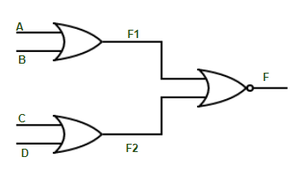OR-NOR Implementation

The outputs of first-level logic gates: F1=A+B and F2=C+D. These outputs are applied as inputs of the second level, so the output of the second level is F=(F1+F2)’ which means F=(A+B+C+D)’.

### NAND-NOR implementation:

The outcome function of NAND-NOR in two-level logic is AND logic. The following is its expression and schematic: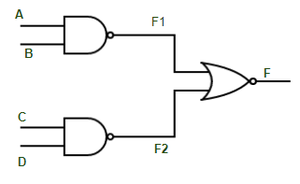NAND-NOR Implementation

The outputs of first level logic gates: F1=(AB) ‘ and F2=(CD)’. These outputs are applied as inputs of the second level, so the output of the second level is F=(F1+F2)’ which means F=((AB)’+(CD)’)’.

### NOR-NAND implementation:

Because the NOR-NAND combination also produces an OR function, it is likewise a degenerate form. The following is an example of it with a diagram;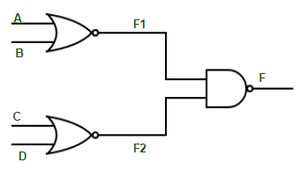NOR-NAND Implementation

The outputs of first level logic gates: F1=(A+B) ‘ and F2=(C+D)’. These outputs are applied as inputs of the second level, so the output of the second level is F=(F1.F2)’  which means F=((A+B)'(C+D)’)’.

### NAND-OR implementation:

This combination, like the AND-NAND combination, produces a NAND logic function.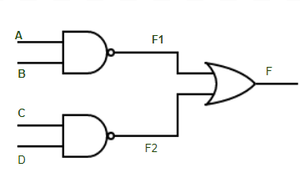NAND-OR Implementation

The outputs of first level logic gates: F1=(AB) ‘ and F2=(CD)’. These outputs are applied as inputs of the second level, so the output of the second level is F=(F1+F2) which means F=((AB)’+(CD)’)=(ABCD)’.

### NOR-AND implementation:

This combination is identical to the OR-NOR combination since this combination similarly results in a NOR function.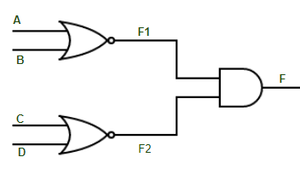NOR-AND Implementation

The outputs of first level logic gates: F1=(A+B) ‘ and F2=(C+D)’. These outputs are applied as inputs of the second level, so the output of the second level is F=(F1.F2) which means F=((A+B)'(C+D)’)=(A+B+C+D)’.

### Non-Degenerative form:

A non-degenerative form occurs when the output of a two-level logic realization cannot be achieved using a single logic gate. Non-degenerate forms are two-level logic combinations that implement the Sum of Product form or the Product of Sum form.

In those 16 combinations, there are 8 non-degenerate forms. Below are instances of each of these non-degenerate types.

### AND-OR implementation:

The first level gate in a While-OR combination is an AND gate, and the second level gate is an OR gate. As shown in the diagram below, this combination implements the Sum of Product (SOP) form.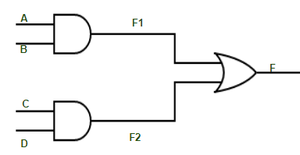AND-OR Implementation

The outputs of first-level logic gates: F1=AB and F2=CD. These outputs are applied as inputs of the second level, so the output of the second level is  F=(F1+F2) which means F=(AB+CD).

### NAND-NAND implementation:

NAND is a universal gate, and its NAND-NAND combination, like the AND-OR combination, is used to produce the Sum of Product form.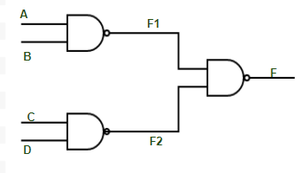NAND-NAND Implementation

The outputs of first-level logic gates: F1=(AB)’ and F2=(CD)’. These outputs are applied as inputs of the second level, so the output of the second level is F=(F1.F2)’ which means F=(AB)+(CD).

### OR-AND implementation:

The first level gate in an OR-AND combination is an OR gate, and the second level gate is an AND gate. The Product of Sum form is implemented with an OR-AND combination.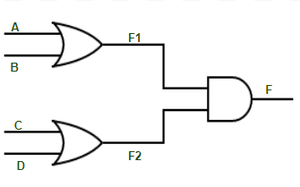OR-AND Implementation

The outputs of first-level logic gates: F1=(A+B) and F2=(C+D). These outputs are applied as inputs of the second level, so the output of the second level isF=(F1.F2) which means F=(A+B)(C+D).

### NOR-NOR implementation:

NOR is also a universal gate and its NOR-NOR combination can be used to implement the Product of Sum form.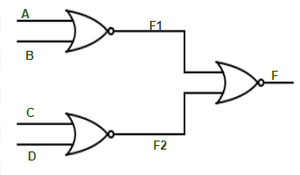NOR-NOR Implementation

The outputs of first-level logic gates: F1=(A+B)’ and F2=(C+D)’. These outputs are applied as inputs of the second level, so the output of the second level isF=(F1+F2)’ which means F=((A+B)’+(C+D)’)’=(A+B)(C+D).

### AND-NOR implementation:

The AND-NOR combination is used to implement the AND-OR-INVERT compound logic (AOI).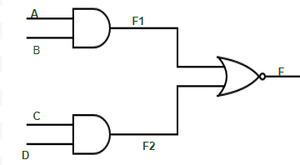AND-NOR Implementation

The outputs of first-level logic gates: F1=(AB) and F2=(CD). These outputs are applied as inputs of the second level, so the output of the second level isF=(F1+F2)’ which means F=(AB+CD)’.

### NAND-AND implementation:

The AND-OR-INVERT (AOI) form can also be implemented using NAND-AND.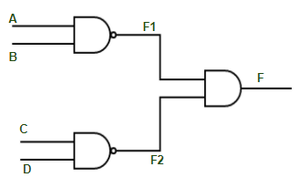NAND-AND Implementation

The outputs of first-level logic gates: F1=(AB)’ and F2=(CD)’. These outputs are applied as inputs of the second level, so the output of the second level isF=(F1F2) which means F=(AB)'(CD)’=(AB+CD)’.

### OR-NAND implementation:

The OR-NAND form is used to implement the OR-AND-INVERT compound logic (OAI).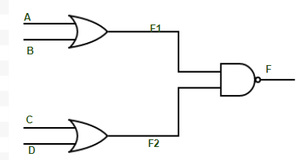OR-NAND Implementation

The outputs of first-level logic gates: F1=(A+B) and F2=(C+D). These outputs are applied as inputs of the second level, so the output of the second level isF=(F1F2)’ which means F=[(A+B)(C+D)]’.

### NOR-OR implementation:

The NOR-OR combination, like the OR-NAND combination, is used to build OR-AND-INVERT compound logic (OAI).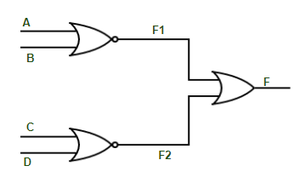NOR-OR Implementation

The outputs of first-level logic gates: F1=(A+B)’ and F2=(C+D)’. These outputs are applied as inputs of the second level, so the output of the second level isF=(F1+F2),  which means F=(A+B)’+(C+D)’=[(A+B)(C+D)]’.

My Personal Notes arrow_drop_up